S k i l l
i n
A R I T H M E T I C

Lesson 9

# MULTIPLY WHOLE NUMBERS MULTIPLY DECIMALS

In this Lesson, we will answer the following:

1. How do we multiply by a single digit?
2. In writing, how do we multiply whole numbers?
3. How do we multiply decimals?
4. How can we find a percent of a number?
5. How can we find the area of a rectangle?

Let us face facts.  The student will quickly replace written multiplication with a calculator.  (What if you don't have a calculator?  What if you don't have a pencilLesson 8.)  Therefore, what should the student know about written multiplication that deserves to be called educational?  The student should know that it is based on the distributive property.  The student should also know the basics of placing the decimal point.

First:

 1. How do we multiply by a single digit?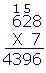Align the multiplier (on the bottom) with the ones digit of the multiplicand, and draw a line. Then multiply each digit of the multiplicand. Write the ones digit of each product below the line. If there is a tens digit, carry it -- add it -- to the next product.

"7 times 8 is 56."  Write 6, carry 5.

"7 times 2 is 14, plus 5 is 19."  Write 9, carry 1.

"7 times 6 is 42, plus 1 is 43."  Write 43.

We can analyze this as follows: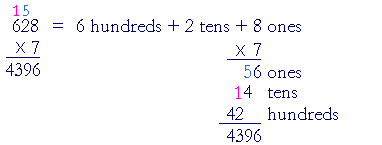7 has been distributed to each unit of 628:  to

6 hundreds + 2 tens + 8 ones.

On the left (but compare the right):

7 × 8 ones = 56 ones, or simply 56.  Write 6 below the line and carry the 5 onto the tens column, because the 5 is 5 tens.

Next:  7 × 2 tens = 14 tens, plus 5 are 19 tens.  Write 9 and carry the 1 onto the hundreds column -- because 19 tens = 190.  The carried 1 is 1 hundred.

Finally:  7 × 6 hundreds = 42 hundreds, plus 1 is 43 hundreds. Write 43.

When the multiplier has more than one digit --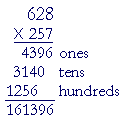-- follow the same procedure for each digit.  However, when we multiply by 5 tens, the product is 3140 tens.  Therefore we write 0 in the tens column.

When we multiply by 2 hundreds, the product is 1256 hundreds, and so we write 6 in the hundreds column.

We can state the rule as follows:

 2. In writing, how do we multiply whole numbers?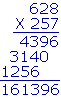Write the multiplier under the multiplicand and draw a line. Multiply the multiplicand by each digit of the multiplier. Place the ones digit of each partial product in the same column as the multiplying digit. Then add the partial products.

Anticipating the next Question, if there were decimal points --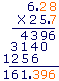-- the multiplication would proceed in exactly the same way. In the answer, we would then separate as many decimal places as there are in the two numbers together; in this case, three.

 Example 1. 96 × 37 672 ← 7 × 96 = 630 + 42 2884 ← 3 × 96 = 270 + 18 3552

On multiplying by 7 ones, write 2 in the ones column.

On multiplying by 3 tens, write 8 in the tens column -- because the partial product 288 means  288 tens.

Rather than do the traditional method of "carrying," the student should be able to calculate each partial product mentally by distributing from left to right.  We have indicated this above.

Example 2.   0's within the multiplier.

 907 × 308 7256 272156 279356
 8 × 907 = 7200 + 56 = 7256 0 × 907 = 0 3 × 907 = 2700 + 21 = 272150

On distributing 8 ones, write 6 in the ones column.

Any number times 0 is 0, therefore it is not necessary to write any digit in the tens column.

On distributing 3 hundreds, write 1 in the hundreds column.

It is not necessary to write rows of 0's.  They add nothing to the product.

 3. How do we multiply decimals? .2 × 6.03 Ignore the decimal points -- do not align them -- and multiply the numbers as whole numbers. Then, starting from the right of the product, separate as many decimal places as there are in the two numbers together.

Example 3.   .2 × 6.03

Solution.  Ignore the decimal points.  Simply multiply

2 × 603 = 1206

Now we must put back the decimal points.  Together,  .2 and 6.03 have three decimal places.  (Lesson 3, Question 4.)  Therefore, starting from the right, separate three places:

1.206

When we ignore a decimal point, we have in effect moved the point to the right:

6.03 → 603

We have multiplied by a power of 10. (Lesson 3, Question 2.)  Therefore, to compensate and name the right answer, we must divide by that power, we must separate the same number of decimal places.

Example 4.   .03 × .002

Solution.   Ignore the decimal points.

3 × 2 = 6

Together, .03 and .002 have five decimal places.  Therefore, separate five places:

.00006

Example 5.   200 × .012

Solution.  Ignore the decimal point.  Multiply

200 × 12 = 2400

Again, to multiply whole numbers that end in 0's, first ignore the 0's, then replace them.  (Lesson 8, Question 2.)  But replace only the 0's on the end of whole numbers.  Do not replace the 0 of .012

Now separate three decimal places (.012):

2.400 = 2.4

How can we find a percent of a number?

8% of \$400 = 8 × \$4 = \$32.

How much, then, is

6% of \$75?

Again, we can analyze as follows:

1% of \$75 is \$.75.

Therefore, 6% of \$75 = 6 × \$.75.

6 × 75 = 420 + 30 = 450

On separating two decimal places (.75):

6% of \$75 = \$4.50

Example 6.   How much is 9% of \$84?

Solution.   Simply multiply 9 × 84, then separate two places.

9 × 84 = 720 + 36 = 756

Therefore, 9% of \$84 = \$7.56.

Example 7.   How much is 3% of \$247?

Solution.   3 × 247 = 600 + 120 + 21 = 741.

Therefore, 3% of \$247 = \$7.41

Example 8.   How much is 11% of \$76?

Solution.   11 × 76 = 760 + 76 = 836

Therefore, 11% of \$76 = \$8.36.

These are simple problems that do not require a calculator.  For more such simple problems, see Lesson 28.  To learn how to do percent problems with a calculator, see Lesson 13.

Area of a rectangle

What is "1 square foot"?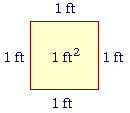1 square foot is a square figure in which each side is 1 foot.

We abbreviate "1 square foot" as 1 ft².

Now here is a rectangle whose base is 3 cm and whose height is 2 cm.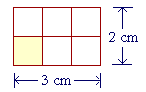What do we call the small shaded square?

Since each side is 1 cm, we call it "1 square centimeter."  And we can see that the entire figure is made up 2 × 3 or 6 of them!

In other words, the area of that rectangle -- the space enclosed by the boundary -- is 6 square centimeters:  6 cm².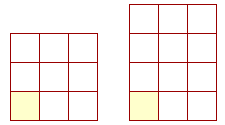If the rectangle were 3 by 3 -- that is, if it were a square -- then it would be made up of 9 cm².  If it were 3 by 4, the area would be 12 cm². And so on.  In every case, to calculate the area of a rectangle, we multiply the base times the height.

Area = Base × Height

When the length is measured in centimeters, the area is measured in square centimeters:  cm².  And similarly for any unit of length.

We have illustrated this with whole numbers, but it will be true for any numbers.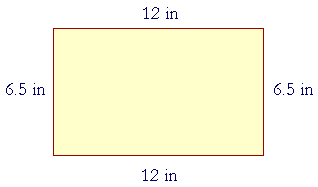If the base is 12 in, and the height is 6.5 in, then to find the area, multiply

12 × 6.5.

Now,

12 × 65 = 650 + 130 = 780

Therefore on separating one decimal place (6.5):

Area = 78 in²

Please "turn" the page and do some Problems.

or

Continue on to the Next Lesson.

www.proyectosalonhogar.com